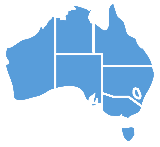Keywords: division, divide

## Sign Definition

### As a Noun

1. The mathematical process of dividing one number by another. English = division.
2. The mathematical symbol for the process of dividing one number by another.

### As a Verb or Adjective

1. In mathematics, to calculate how many times a small number can go into a large number. English = divide.